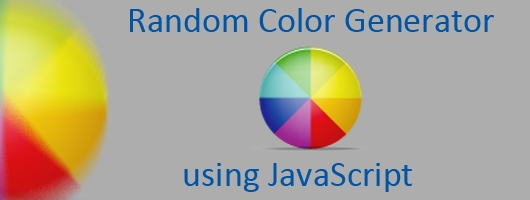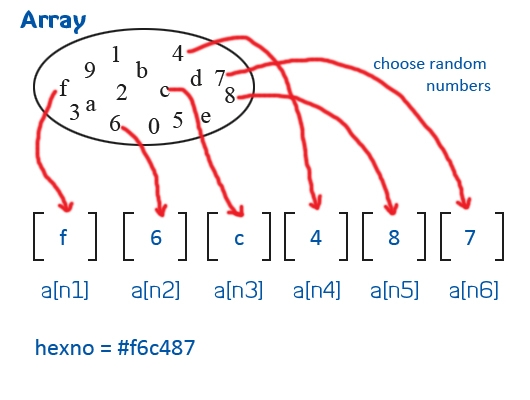# Create Simple Random Color Generator using JavaScriptHello everyone! I am writing a post after long time since I was busy in 12 hours a day tight schedule for almost Six months. Although the experience was very awesome.

So today, we are going to create a very simple Random Color Generator using JavaScript. Some of you guys might think it as very very simple but it’s really fun to create. If you haven’t created yet by yourself then I would suggest trying it. It actually takes 2-3 minutes for creating.

Here we are going to generate Hex Number. For this we will create an Array which will contain numbers ‘0’ to ‘9’ and characters ‘a’ to ‘f’ as the range of the Hex Number is from 0x000000 to 0xFFFFFF. Then we will pick an element from an array randomly and place it in the Hex Number.### Step1:

Create an Array
[javascript]

var hexno=new String;
arr=new Array(“0″,”1″,”2″,”3″,”4″,”5″,”6″,”7″,”8″,”9″,”a”,”b”,”c”,”d”,”e”,”f”);

[/javascript]

### Step 2:

We will select an element randomly from array. Math.random() generates random number from 0 to 1 which will be multiplied by 15 (since our array contains 16 elements with base 0). Math.round() will round the number to its nearest natural number.
[javascript]

var n1=Math.round(Math.random()*15);

var n2=Math.round(Math.random()*15);

var n3=Math.round(Math.random()*15);

var n4=Math.round(Math.random()*15);

var n5=Math.round(Math.random()*15);

var n6=Math.round(Math.random()*15);

[/javascript]

### Step 3:

Get a corresponding number for all the six elements and put it in the string.
[javascript]

hexno=”#”+arr[n1]+””+arr[n2]+””+arr[n3]+””+arr[n4]+””+arr[n5]+””+arr[n6];

[/javascript]

### Step 4:

Use the string to apply the color.
[javascript]
window.document.bgColor=hexno;
[/javascript]

That’s it. Random Color Generator is ready. Try it out.
Final Code:
[javascript]
var hexno=new String;
arr=new Array(“0″,”1″,”2″,”3″,”4″,”5″,”6″,”7″,”8″,”9″,”a”,”b”,”c”,”d”,”e”,”f”);

var n1=Math.round(Math.random()*15);
var n2=Math.round(Math.random()*15);
var n3=Math.round(Math.random()*15);
var n4=Math.round(Math.random()*15);
var n5=Math.round(Math.random()*15);
var n6=Math.round(Math.random()*15);

hexno=”#”+arr[n1]+””+arr[n2]+””+arr[n3]+””+arr[n4]+””+arr[n5]+””+arr[n6];

document.write(“<div id=’tag’>”);

document.write(“<h2>Hex Color</h2>”+”<h3>”+hexno+”</h3>”);

document.write(“</div>”);

window.document.bgColor=hexno;
[/javascript]